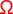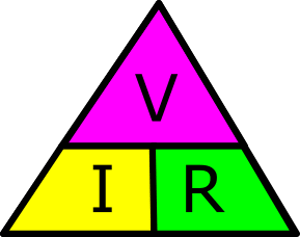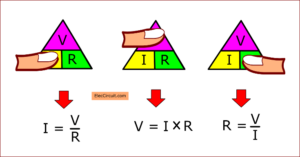# Relationship voltage current resistance and Ohms Law

Did you use a 1.5V battery? What is a voltage? You know its voltage, 1.5V. If you are a beginner. Do you understand the Relationship between voltage, current, and resistance?  Someone may not understand them. Also, the Ohms Law learning is basic electronics that easy.

Electricity is the ordered flow of electrons in a conductor.

When having electricity always has voltage, current, and resistance. In the block diagram below.

You can imagine them like the water in a large tank.

• The height of a water tank from the ground is like the voltage
• The amount of water is like the current.
• The water pipe is like a resistance

You are not worried if you not understand Relationship between voltage current and resistance. I believe that if you look at the next image, you will understand more.

## Meet the current

The current is the quantity of electrons passing a point. The unit of current is the Ampere. Or in easier! It is like water volume. We can measure it in Amps, A.

When you have a many water volume, you can use the water in longer. It looks like this.

If you have a battery with a large capacity for electricity. So, you can use the battery for longer.

For example, 12V 10Ah battery can use for longer than 12V 5Ah battery at the same load.

## Meet the Voltage

You often see it in various positions in general.

For example, a battery, AC main, Behind the appliance, etc.

The voltage is the pressure of electrical. The voltage drop is the difference in voltage between the two ends of a conductor through with current is flowing.

We can measure it in volts, V or E.

In the easier way!

The current is likes the water flowing through a pipe.  Then, the voltage is the water pressure.

The voltage looks like water pressure in different height.   We put the water tank at a high level. It makes the water pressure much stronger.   The water can flow longer distances.

Also, high voltage can pressure the current well.

## Meet power

The power is a performed work by an electrical current. The use of power is the watts, W. The power of the direct current (DC) or energy is the voltage multiplied by current.  We often this in any time, behind the appliance, or light bulb etc.

For example, the lamp 10W 12V. So, its power is 10 watts at 12V. Do you wonder how much the electrical current it uses?  Please below in the ohms law.

## Meet resistance

The most conductors are not perfect. They have a resistance. When the voltage pushes the current in conductors. The resistance inside the conductor will resist the flow of current.  We can measure or read it in the ohms,.

Then, we may compare it likes the water pipe. The size of the pipe affects the amount of water flowing out.

• The large pipe likes low resistance. The current can flow a lot.
• The small pipe likes a high resistance. Then, the current can flow a fewer.

## Ohm’s Law in basic uses

This law is a relationship of voltage, current, resistance, and power. Which we can find these formulas known as Ohm’s Law:

• V  = I x R — “V” is “I” multiplied by “R”.
• I  = V / R — “I” is “V” divided by “R”.
• R  = V / I — “R” is “V” divided by “I”.
• P  = V x I — “P” is “V” multiplied by “I”.

From the example
How many currents the does lamp use?
P = V x I
So: I = P / V ; P = 10W , V = 12V.
I = 10W / 12V
= 0.83A

If you want to use it for 2 hours.
You need to use a 12V battery that can power more than 2Ah up.

When I was a kid. I cannot remember this Ohm’s Law formulas. I have used the Ohms Law Triangle.How to use Ohms law triangle
Suppose I want to know the current that the lamp is using. So I closed my finger at “I”.

We find the current with Ohms law triangle

Then, we can get the current(I) from Ohm’s law.

I = V/R

V is the voltage of the battery, 12V.
R is the resistance of the lamp. Which I measure its resistance to be about 10 ohms.

So the current is.

I = 12V / 10 ohms
= 1.2A

Thus, the current that the lamp is about 1.2A.

You will see that, we can find the current, voltage and resistance with Ohms law triangle.The Power Triangle
You can find the power with the power triangle in the same way as above.
P = I x V
or
I = P / V
or
V = P / I

If you look in the block diagram below. You will understand better.

The Finding power, current, and  voltage with the power Ohms law triangle triangle

For example:
Suppose you have a DC power supply, 12V 2A output. Then, your friend ask you. What watts is it?

In block diagram, P = V x I
V = 12V : I = 2A
So its power is.
P = 12V x 2A
= 24 watts.

It can use it for 20 watts of lamp.

All of this is suitable for basic level learners. You can use a normal calculator to find the necessary values.

Next Step:
Quick Common Electronics Components

Get Ebook: Simple Electronics Vol-03

### Related Posts

We always try to make Electronics Learning Easy.

### 5 thoughts on “Relationship voltage current resistance and Ohms Law”

1. It is interesting to meet like minds like you, always enjoy this platform.

• Hi Victor
Thanks for the feedback. I hope my post has useful for you.